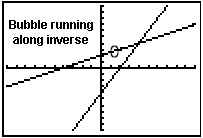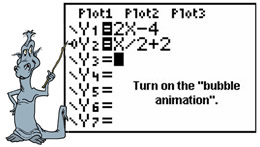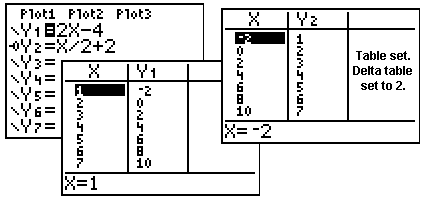Inverse Functions

The graphing calculator can be used in several ways to check your work
when you are dealing with inverse functions.

1.
 Use the calculator to quickly determine if the inverse of your graph is a function.

Graph the original function and observe the results of the horizontal line test

 Example:  Is the inverse of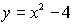a function? Since this parabola does not pass the horizontal line test , the inverse will not be a function.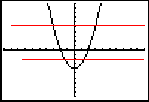The red lines will not appear on the calculator.

2.
 Use the calculator to determine if your algebraic inverse answer is correct. Method 1:
A quick way to determine if your algebraic inverse answer is correct is to:
a.)  enter your starting function in Y1.
c.)  enter Y1(Y2) in Y3. (composition of functions)
d.)  GRAPH
e.)  if Y3 produces the graph of the identity function, y = x, your algebraic inverse answer is correct.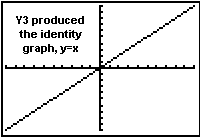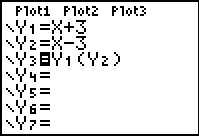Use the calculator to determine if your algebraic inverse answer is correct. Method 2:
The graphing calculator has a DrawInv command that will DRAW a function's inverse.  To use this feature, place the original starting function into Y1.  Press  2ndPRGM (DRAW) #8 DrawInv.  The command will appear on the home screen waiting for a parameter.  Enter the location of the function to be investigated, Y1.   The graph of the inverse will appear.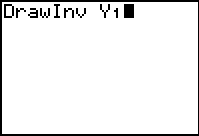If you wish to use this feature to check an algebraic answer,
a.  Enter the original function into Y1.
 d.  Enter your algebraic answer for the     inverse into Y2  with the bubble animation (in front of Y2), and hit GRAPH.  e.  If your algebraic answer is correct, the bubble animation will follow the inverse graph drawn by the calculator.